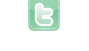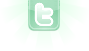# Greyscaling an image

```   for (int x = 0; x < getWidth(); x++)
{
for (int y = 0; y < getHeight(); y++)
{
GreenfootImage bg = getBackground();
Color beep = bg.getColorAt(x,y);
Color gray = new Color(beep.getRed(),beep.getGreen(),beep.getBlue());

}
}```
Trying to make an image gray like an old photo, but I'm stuck. This is the code I have so far, where do I go from here?
```for (int x = 0; x < getWidth(); x++)
{
for (int y = 0; y < getHeight(); y++)
{
GreenfootImage bg = getBackground();
Color beep = bg.getColorAt(x,y);
int red = beep.getRed()/2;
int green = beep.getGreen()/2;
int blue = beep.getBlue()/2;
int grey =
Color gray = new Color(,,);

}
}```
Slight update
LucarioCodes wrote...
Trying to make an image gray like an old photo, but I'm stuck. This is the code I have so far, where do I go from here?
Not tested, but I believe if you just add the three color parts together and divide by 3, then use that value for all three parts should be close to what you want:
```int grey = (beep.getRed()+beep.getGreen()+beep.getBlue())/3;
Color gray = new Color(grey, grey, grey);```